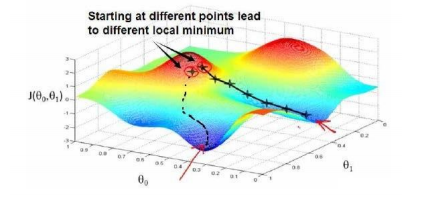# Kami third home

Personal blog

### 梯度下降算法• := -表示赋值
• $\alpha$ -学习率，它决定了我们沿着能让代价函数下降程度最大的方向向下迈出的步子有多大。α越小，步长越小，α越大，步长越大
• $\frac{\partial}{\partial\theta_j}J(\theta_0,\theta_1)$-梯度

$\theta$赋值，使得$J(\theta)$按梯度下降最快的方向进行，一直迭代下去，最终得到局部最小值。$\alpha$是学习率，它决定了我们沿着能让代价函数下降程度最大的方 向向下迈出的步子有多大。

j=0时：

j=1时: